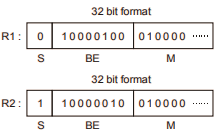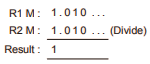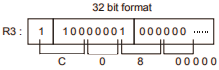# GATE Solved Paper 2020 - GATE 2020

• A

I and IV only• B

I and II only• C

III and IV only• D

II and III only• Option : D
• Explanation :
d(AB) = d(A) × d(B)
d(A + B) ≥ d(A) + d(B)
r(A + B) ≤ r(A) + r(B)
r(AB) ≠ r(A) r(B)

• A

Θ(log n + k)• B

Θ(n log k)• C

Θ(log n)• D

Θ(k log n)• Option : A
• Explanation :To find a number between range [x, y] requires log n comparisons.
As, K numbers are to be found, K + log n would be time complexity.

• A

0×83400000• B

0×C0800000• C

0×40800000• D

0×C8500000• Option : B
• Explanation :
R1: 0x42200000
R2: 0xC12000001. Subtract the exponents i.e. divisor exponent from the dividend exponent.2. Divide the mantissa i.e3. Sign (opposite sign division result sign is –ve)Hence, option (B) is correct answer.

 T1 RA RC WD WB Commit T2 RB WB RD WC Commit
• Option : D
• Explanation :
R2(B) is conflicting with W1(B) so, W1(B) should always come after R2(B)
W2(B) is conflicting with W1(B) similarly, W1(B) should always come after W2(B)
R2(D) is conflicting with W1(D), so, W1(D) should always come after R2(D)
R1(C) is conflicting with W2(C) so, W2(C) should always come after R1(C)
Therefore the transaction will be:  T1 T2 R(B) W(B) R(D) R(A) R(C) W(D) W(B) W(C) Commit Commit

• Option : B
• Explanation :
R has a nontrivial functional dependency X → A where X is not a sup and A is a prime attribute.
Related Quiz.
GATE 2020